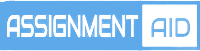# Mathematical statistics help#### Mathematical Statistics

What is mathematical statistics?

Statistics is a study of mathematical science that involves data collection, organization, analysis, interpretation and presentation. Statistics is a tool used for prediction and forecasting through the use of data and statistical models. It is broadly divided into two fields; Descriptive statistics and inferential statistics. In statistics you get to encounter topics like probability distributions, Bernoulli distribution, regression and many more.

So, what do we mean by mathematical statistics? The application of mathematics to statistics  is called mathematical statistics. It is a branch of statistics, a mathematical point of view based on the theory of probability and some mathematical concepts such as linear algebra and analysis. It includes the derivation of some theorems related to;

1. Basic probability theory

2. Random variables

3. Probability distributions

4. Normal distributions and

5. Associated distributions.

In particular, mathematical statistics mainly deals with the basic concepts of statistical theory such as likelihood functions, sufficient statistics, and exponential family of distributions. More to it, it clearly derives the theorems and proofs related to the concepts of point estimation, minimum variance unbiased estimate (MVUE), Cramer-Rao inequality, Maximum likelihood estimates and asymptotic theory. Therefore, this statistical branch can be said to be the fundamental of all advanced concepts dealt with in statistical theory.

Applications of mathematical statistics, mathematical statistics with applications.

Mathematical statistics have found great use in areas of research and interest including;

1. Health and medicine mainly in the study of disorders and treatments like in the analyzing  the efficacy of the latest treatment measures, how these are better than traditional curative measures etc.

2. Biology; used in biological research to create models and conduct analysis of everything, from small organisms to complex bio-systems.

3. Weather; Partial differential equations are used by mathematicians to model the atmosphere, oceanic movement, weather pattern etc, and even analyze how to harness tide and wind power. It can also be used to analyze the huge amount of information already present about such systems, and check whether there are any patterns.

4. Genetics; plays an important part in genetics research, in DNA modeling, its replication and functions

5. Industrial processes; used in industrial modeling for studying issues in goods production and distribution and the delivery of services

Mathematical statistics help

Statistics is a very complex subject with lots of concepts involved which many students find it difficult to understand as well as apply them. Hence they mostly end up getting poor grades in their mathematical statistics assignments, mathematical statistics projects or thesis and even in their mathematical statistics exams. This not only affects their overall score but also affects the future career prospects of the student. It is following this that most students end up seeking for Mathematical statistics assignment help or Mathematical statistics homework help looking for tutors to aid in their concept understanding or professional writers to handle their assignments. . If looking for any assistance, consider our  Mathematical statistics assignment help where we offer reliable online homework help at an affordable rate.

## Example Samples

live:topwrit

###### Email:

support@assignmentsaid.com

###### Open Hours:

Mon-Sat: 9am - 6pm